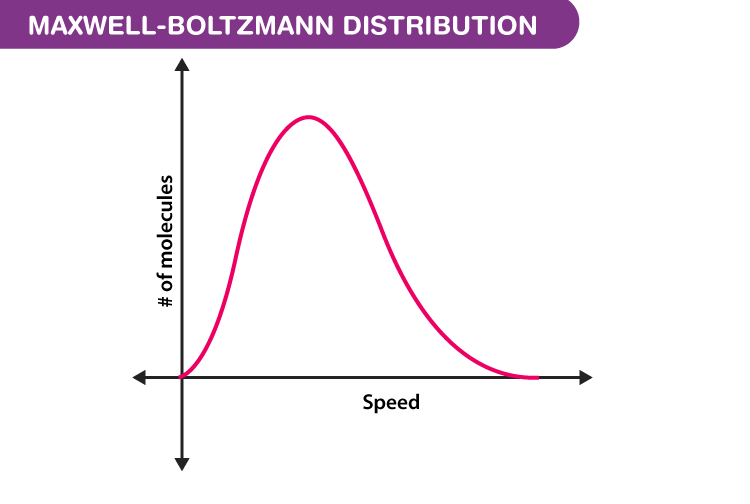# Maxwell Boltzmann Distribution Derivation

Not all the air molecules surrounding us travel at the same speed. Some air molecules travel faster, some move with moderate speeds, and some air molecules will hardly move at all. Hence, instead of asking about the speed of any particular gas molecule, we ask for the distribution of speed in a gas at a certain temperature. James Maxwell and Ludwig Boltzmann came up with a theory to show how the speeds of the molecule are distributed for an ideal gas. The distribution is often represented using the following graph.In the next section, let us look at the Maxwell Boltzmann distribution derivation.

## Derivation of Maxwell-Boltzmann Distribution

Consider a system that consists of identical yet distinguishable particles.

Let the total number of particles in the system be ‘n’.

The total volume of the system is fixed and is given by ‘V’.

The total amount of the energy is fixed and is given by ‘U’.

We, now, want to know the number of particles at a given energy level.

The energy levels available within the system are given by $$\varepsilon _0,\varepsilon _1,\varepsilon _2……….,\varepsilon _r$$. The energy levels are fixed for the system.

The number of particles sitting at each energy level is variable and the number of particles is given by $$n_1,n_2,n_3……..,n_r$$.

The number of ways to attain a given microstate is given by the formula

$$\omega =\frac{n!}{n_{0}!n_1!n_{2}!….n_{r}!}$$ (1)

We need to know the combination of n1,n2,n3 up to nr that maximizes the value of $$\omega$$. This combination results in the most probable microstate, if it is a most probable state then it can be considered as the equilibrium state. To find the combination, let us alter eq (1) as follows

$$\ln \omega =\frac{\ln n!}{\coprod_{i=0}^{i=r}=n_{i}!}$$ (2)

To maximise the value of $$\omega$$, instead of dealing with $$\omega$$ let us deal with $$\ln \omega$$.

$$\ln \omega=\ln n!-\sum_{i=0}^{i=r}\ln n_i!$$ (3)

According to Sterling’s approximation, the log of factorial can be approximated as

$$\ln x!=x\ln x-x$$

Making use of the Stirling approximation in eq (3), we get

$$\ln \omega =n\ln n-n-\sum_{i=0}^{i=r}[n_{i}\ln{n_i}-n_i]$$

By taking the derivative of the above equation, we get

$$\partial \ln \omega=-\sum_{i=0}^{i=r}\partial n_{i}\ln n_{i}+n_{i}\times \frac{1}{n_{i}}-\partial n_{i}=0$$

The above equation implies that

$$\sum_{i=0}^{i=r}[\partial n_i\ln (n_i)]=0$$ (4)

As we know that n is a constant, therefore we can say that

$$\sum_{i=0}^{i=r}n_i=n=constant$$

This means that

$$\sum_{i=0}^{i=r}\partial n_i=0$$ (5)

The sum of all the changes in the system should sum up to zero.

The total energy of the system should also be constant.

$$\sum_{i=0}^{i=r}\varepsilon _i n_i=U=constant$$

Differentiating the above equation, we get

$$\sum_{i=0}^{i=r}\varepsilon _i \partial n_i=U=0$$ (6)

To maximise the function (4) subjected to constraints (5) and (6), let us use the Lagrange’s method of undetermined multipliers.

Multiplying (5) by ɑ and multiplying (6) by 𝛽 and then adding 4, 5 and 6, we get

$$\sum_{i=0}^{i=r}[\ln n_{i}+\alpha +\beta \varepsilon_i]\,\partial{n_i}=0$$ (7)

From eq (7) to be zero, the term $$[\ln n_{i}+\alpha +\beta \varepsilon_i]$$ has to be zero.

$$\ln n_{i}+\alpha +\beta \varepsilon_i=0$$

Simplifying the above equation, we get

$$n_i=e^{-\alpha}\times e^{-\beta\epsilon_i}$$ (8)

The above equation signifies the number of particles in i th level.

In eq (8), the only variable parameter is $$epsilon_i$$, therefore taking the sum, we get

$$\sum_{i=0}^{i=r}n_i=e^{-\alpha}\sum_{i=0}^{i=r} e^{-\beta\epsilon_i}=n$$

Simplifying, we get

$$e^{-\alpha}=\frac{n}{\sum_{i=0}^{i=r} e^{-\beta\epsilon_i}}$$

The above equation is the same as

$$e^{-\alpha}=\frac{n}{P}$$ (9)

where P is the partition function, $$P=\sum_{i=0}^{i=r} e^{-\beta\epsilon_i}$$

Substituting (9) in (8), we get

$$n_i=\frac{n}{P}e^{-\beta \epsilon _i}$$ (10)

The value of 𝛽 in the above equation is $$\beta=-\frac{1}{k_{b}T}$$, where kb is the Boltzmann constant and T is the temperature.

Substituting the value of 𝛽 in eq (10), we get

$$n_i=\frac{n}{P}\,e^{\frac{-\epsilon_i}{k_{B}T}}$$

The above expression helps find the number of particles in the most probable microstate. This expression is known as the Maxwell Boltzmann statistics expression.